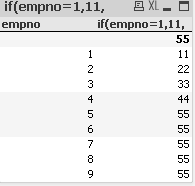# New to QlikView

Discussion board where members can get started with QlikView.

Announcements

#### Breathe easy -- you now have more time to plan your next steps with Qlik! QlikView 11.2 Extended Support is now valid through December 31, 2020. Click here for more information.Not applicable

## Sum of multiple field value

Hi,

how to sum multiple field value into single expression.

=sum({\$<DIVISION ={'L'}>} GROSSWT) OR sum({\$<DIVISION ={'D'}>} PCS)

In above mention example, If I am using first part I am able to see the sum of division "L" on my expression but when I am addting OR for second value my calculation give me -1 as value.

Any idea why ? and if any other way to do that.

1 Solution

Accepted SolutionsMVP

## Re: Sum of multiple field value

Try this,

Sum(if(DIVISION ='L',GROSSWT,if(DIVISION='D',PCS)))

Regards,

Kaushik Solanki

8 RepliesMVP

## Re: Sum of multiple field value

Are you maybe looking for

=sum({\$<DIVISION ={'L'}>} GROSSWT) + sum({\$<DIVISION ={'D'}>} PCS)

or using rangesum

=rangesum(sum({\$<DIVISION ={'L'}>} GROSSWT),sum({\$<DIVISION ={'D'}>} PCS) )

?

OR is a logical operator and will return either -1 (true) or 0 (false)

Hope this helps,

StefanNot applicable

## Re: Sum of multiple field value

wat is the complete expr: any if expr ?MVP

## Re: Sum of multiple field value

Try this.

Sum(if(Wildmatch(DIVISION,'L'),GROSSWT,if(wildmatch(DIVISION,'D'),PCS)))

Regards,

Kaushik SolankiMVP

## Re: Sum of multiple field value

Try this,

Sum(if(DIVISION ='L',GROSSWT,if(DIVISION='D',PCS)))

Regards,

Kaushik SolankiNot applicable

## Re: Sum of multiple field value

Hi

try the below expression

=if(DIVISION='L',sum(GROSSWT),

if(DIVISION='D',sum(PCS),null()))Not applicable

## Re: Sum of multiple field value

Hi

below i give sample exp

if(empno=1,11,

if(empno=2,22,

if(empno=3,33,

If(empno=4,44,55)

)))

in the above exp if empno=1 then it give 11,

for 2 it show 22,for 3 give 33,for 4 give 44

otherwise give 55 for remaining values.

below is result tableNot applicable

## Re: Sum of multiple field value

Thanks to you all.

With below mention expression I was able to get reply.

sum(if(DIVISION='D', PCS,GROSSWT))

New Contributor III

## Re: Sum of multiple field value

Hey,

I am looking for this kind of sum that you have mentioned:

=sum({\$<DIVISION ={'L'}>} GROSSWT) + sum({\$<DIVISION ={'D'}>} PCS)

However, I noticed that the "+" is not summing it up for me. Only one of the two expressions is executed. Do you have any idea on how to sum tow sums??  I already have provided an example it would be great if you could have a look at it. The contribution title is "getting the quarters right"